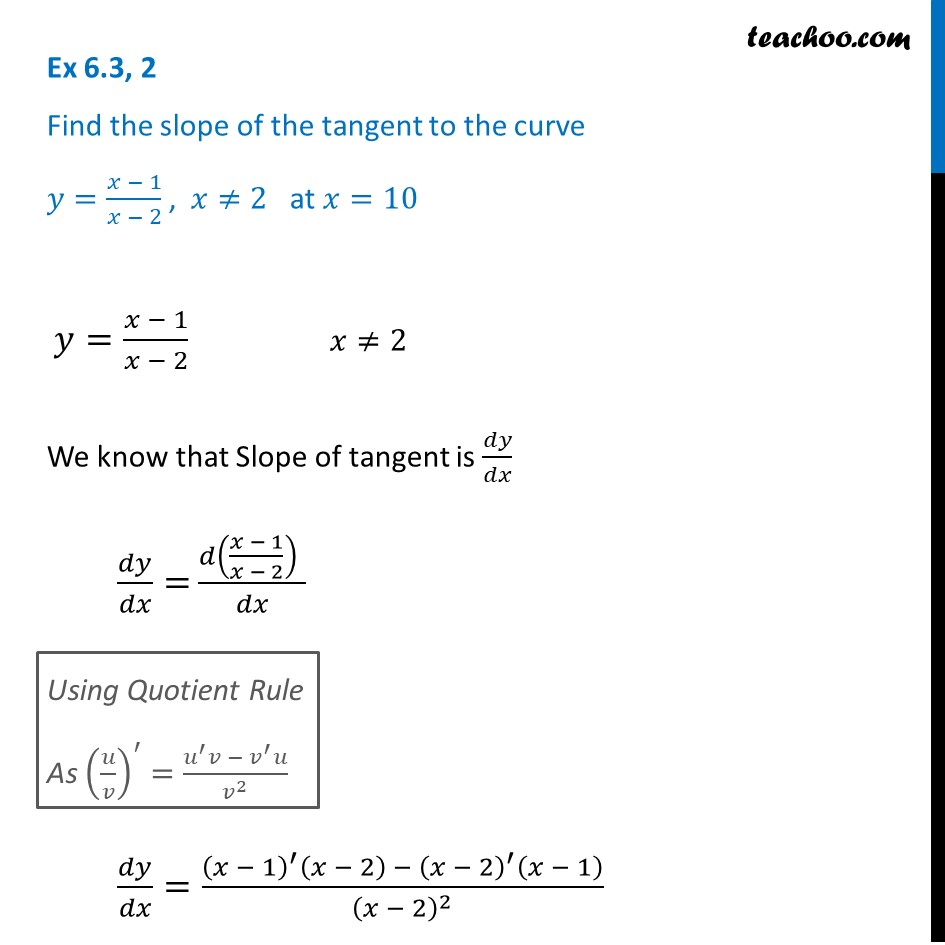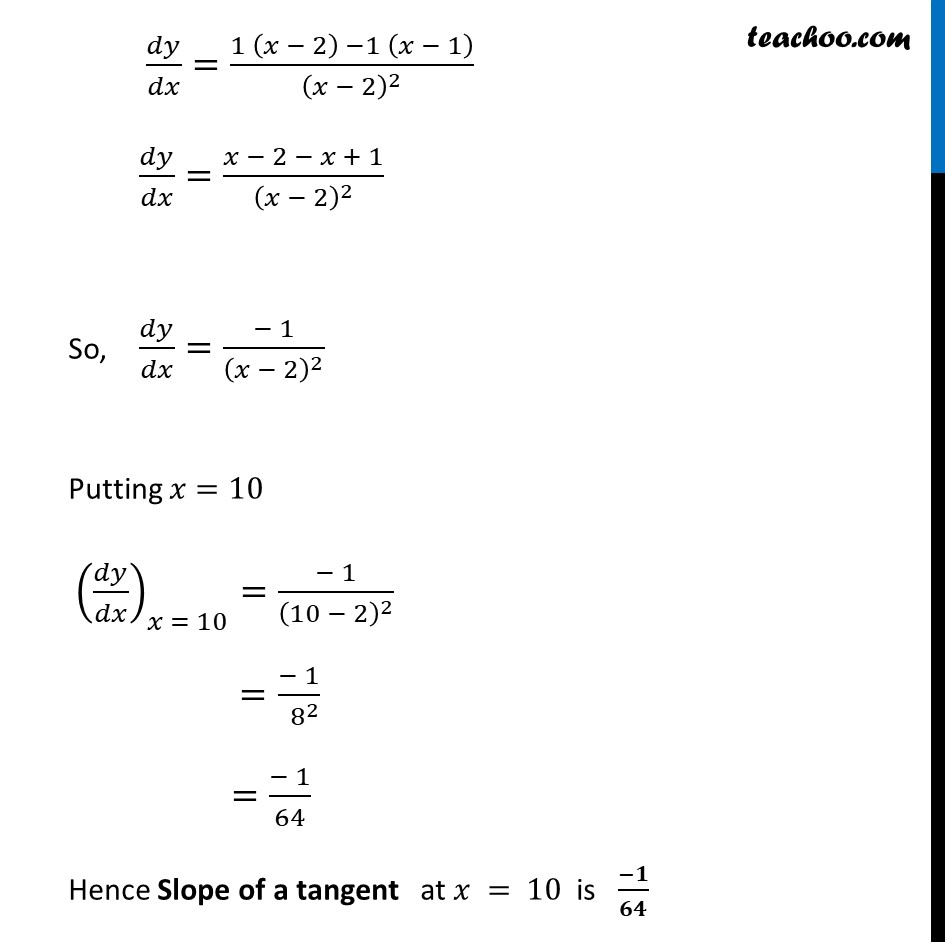Ex 6.3

Chapter 6 Class 12 Application of Derivatives
Serial order wiseMaths Crash Course - Live lectures + all videos + Real time Doubt solving!

### Transcript

Ex 6.3, 2 Find the slope of the tangent to the curve 𝑦=(𝑥 − 1)/(𝑥 − 2) , 𝑥≠2 at 𝑥=10 𝑦=(𝑥 − 1)/(𝑥 − 2) 𝑥≠2 We know that Slope of tangent is 𝑑𝑦/𝑑𝑥 𝑑𝑦/𝑑𝑥= 𝑑((𝑥 − 1)/(𝑥 − 2))" " /𝑑𝑥 𝑑𝑦/𝑑𝑥=((𝑥 − 1)^′ (𝑥 − 2) − (𝑥 − 2)^′ (𝑥 − 1))/(𝑥 − 2)^2 Using Quotient Rule As (𝑢/𝑣)^′=(𝑢^′ 𝑣 −〖 𝑣〗^′ 𝑢)/𝑣^2 𝑑𝑦/𝑑𝑥=(1 (𝑥 − 2) −1 (𝑥 − 1))/(𝑥 − 2)^2 𝑑𝑦/𝑑𝑥=(𝑥 − 2 − 𝑥 + 1)/(𝑥 − 2)^2 So, 𝑑𝑦/𝑑𝑥=(− 1)/(𝑥 − 2)^2 Putting 𝑥=10 (𝑑𝑦/𝑑𝑥)_(𝑥 = 10)=(− 1)/(10 − 2)^2 =(− 1)/〖 8〗^2 =(− 1)/64 Hence Slope of a tangent at 𝑥 = 10 is (−𝟏)/𝟔𝟒